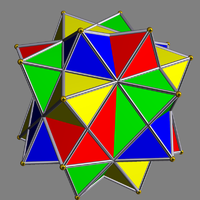# Compound of four octahedra with rotational freedom

﻿
Compound of four octahedra with rotational freedom
Compound of four octahedra with rotational freedomType Uniform compound
Index UC10
Polyhedra 4 octahedra
Faces 8+24 triangles
Edges 48
Vertices 24
Symmetry group pyritohedral (Th)
Subgroup restricting to one constituent 6-fold improper rotation (S6)

This uniform polyhedron compound is a symmetric arrangement of 4 octahedra, considered as triangular antiprisms. It can be constructed by superimposing four identical octahedra, and then rotating each by an equal angle θ about a separate axis passing through the centres of two opposite octahedral faces, in such a way as to preserve pyritohedral symmetry.

Superimposing this compound with a second copy, in which the octahedra have been rotated by the same angle θ in the opposite direction, yields the compound of eight octahedra with rotational freedom.

When θ=0, all four octahedra coincide. When θ is 60 degrees, the more symmetric compound of four octahedra (without rotational freedom) arises. In another notable case (pictured), for a certain intermediate value of θ in which 24 of the triangles form coplanar pairs, the compound assumes the form of the compound of five octahedra with one of the octahedra removed.